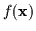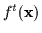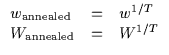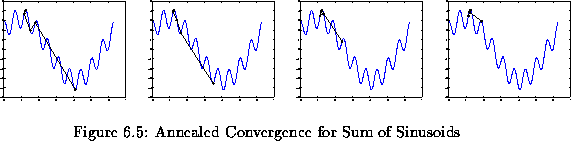Next: High Dimensions Up: Optimization - General Bound Previous: Fourier Decomposition Optimization

# Beyond Local Optimization: Annealing the Bounds

A pivotal property of the above local maximization approach is the fact that it can be extended into a more global maximization algorithm. We shall derive explicit mechanisms for deterministically annealing the optimization such that it converges (asymptotically) to a more global maximum of a function from any local maximum attractor basin. Deterministic annealing has been applied in statistical approaches (i.e. for the EM algorithm ) very successfully and in general is better motivated than the ad hoc approaches associated with gradient ascent (such as momentum or weight decay). Although a detailed derivation and discussion of this mechanism and our usage of it is beyond the scope of this work, we shall discuss some of the implementation and motivation currently being considered.

An important insight can be gleaned from the deterministically annealed joint density estimation approaches and their recent success. Although the annealing approach hasn't quite become a standard mechanism in the learning community, it has been used more and more in very straightforward estimation problems (i.e. Gaussian mixture models mostly). Simply put, annealing in EM-type algorithms involves placing a distribution over the contact point (i.e. current operating point) x*. Thus, the algorithm starts with a relatively flat distribution (corresponding to high temperature) indicating that it does not know where to initialize its local maximum search. Eventually, as the optimization iterates, this distribution gets 'tightened' and narrows towards a more localized x* as the temperature is cooled and the algorithm behaves as a local optimizer. Thus, the optimization is less sensitive to the initialization point and the local maximum basin it happens to start in.

In the spirit of the above annealing process, we can imagine a different type of metaphor. Consider the function we are optimizing (i.e.) which is speckled with many local maxima. Now consider a heavily smoothed version ofwhich we shall call(where t is proportional to the amount of smoothing). By smoothing, imagine flattening out the function and its many little local maxima by convolving it with, say, a Gaussian kernel. Evidently, after enough convolutions or smoothings,will start looking like a single maximum function and more global maximization is possible. Thus, we can run a local optimization scheme onand get to its single global maximum. As we reduce the degree of smoothness,starts to look more and more like the original functionand we will begin optimizing the true function locally. However, the current operating point has now been placed in the basin of a more global maximum. It is important to note that here the term 'global maximum' is somewhat of a misnomer since a small 'peaky' global maximum might get ignored in favor of a wider maximum. This is due to the width of the effective basin around the sub-optimal maximum which has an effect on how dominant it remains after smoothing. The higher a maximum is and the more dominant and smooth the basin that surrounds it, the more likely it will remain after heavy smoothing.

Actually, it could be argued that this peculiarity of the 'smoothing based annealing' here is an advantage rather than a disadvantage. It is well known that robustness (of a maximum) is often a critical feature of optimization and estimation. By robustness we adopt the following definition: a robust maximum is a high maximum which is somewhat insensitive to perturbations in the locus or the parameters. This is similar to the Bayes' risk argument. Thus, the annealing process described here should move the optimization algorithm into a wider basin which is typically more robust. A sharp, peaky true global maximum might be the result of some artifact in the function being optimized (i.e. like overfitting in estimation problems). This kind of abnormality might not be typical of the landscape of the function and therefore should be ignored in favor of a wider more robust maximum.

Of course in practice, explicit smoothing of the functionis a cumbersome task either numerically or analytically. So, what we shall do is smooth the function implicitly. This is done by artificially widening the parabolas that are used to bound. In essence, we are paying more attention to wider parabolas in the optimization. To accomplish this, every w term or W matrix is taken to a power less than one (for higher temperatures, the power factor is closer to zero). This is shown more explicitly in Equation 6.26.(6.26)

Of course, T is a temperature parameter which has to be varied using a pre-determined schedule. We will use the traditional exponential decay schedule which starts the temperature off near infinity and drops it towards T=1. At infinity, the parabolas are effectively flat lines. Thus, the hypothetical function they bound is also flat and has in fact 0 local minima. At T=1 the system reverts to the typical local maximization performance described earlier.

In Figure 6.11 some examples of the annealing are shown on combinations of sinusoids. Almost all the initialization points lead to convergence to the same global maximum. The exponential decay coefficient used was 0.4 and global convergence was obtained in about 5 iterations every time.Effectively, the annealing technique considers local maxima as basins with a volume (in 1D this is just a height and width). The larger (wider and taller) basins will dominate as we smooth the function and force convergence towards a more global solution. Also note that deterministic annealing is quite different from simulated annealing. Only a few steps were required to achieve global convergence. In simulated annealing, random steps are taken to 'skip' out of local maxima in hopes of landing in a better basin. This is typically a less efficient search technique than a deterministic optimization approach.Next: High Dimensions Up: Optimization - General Bound Previous: Fourier Decomposition Optimization
Tony Jebara
1999-09-15